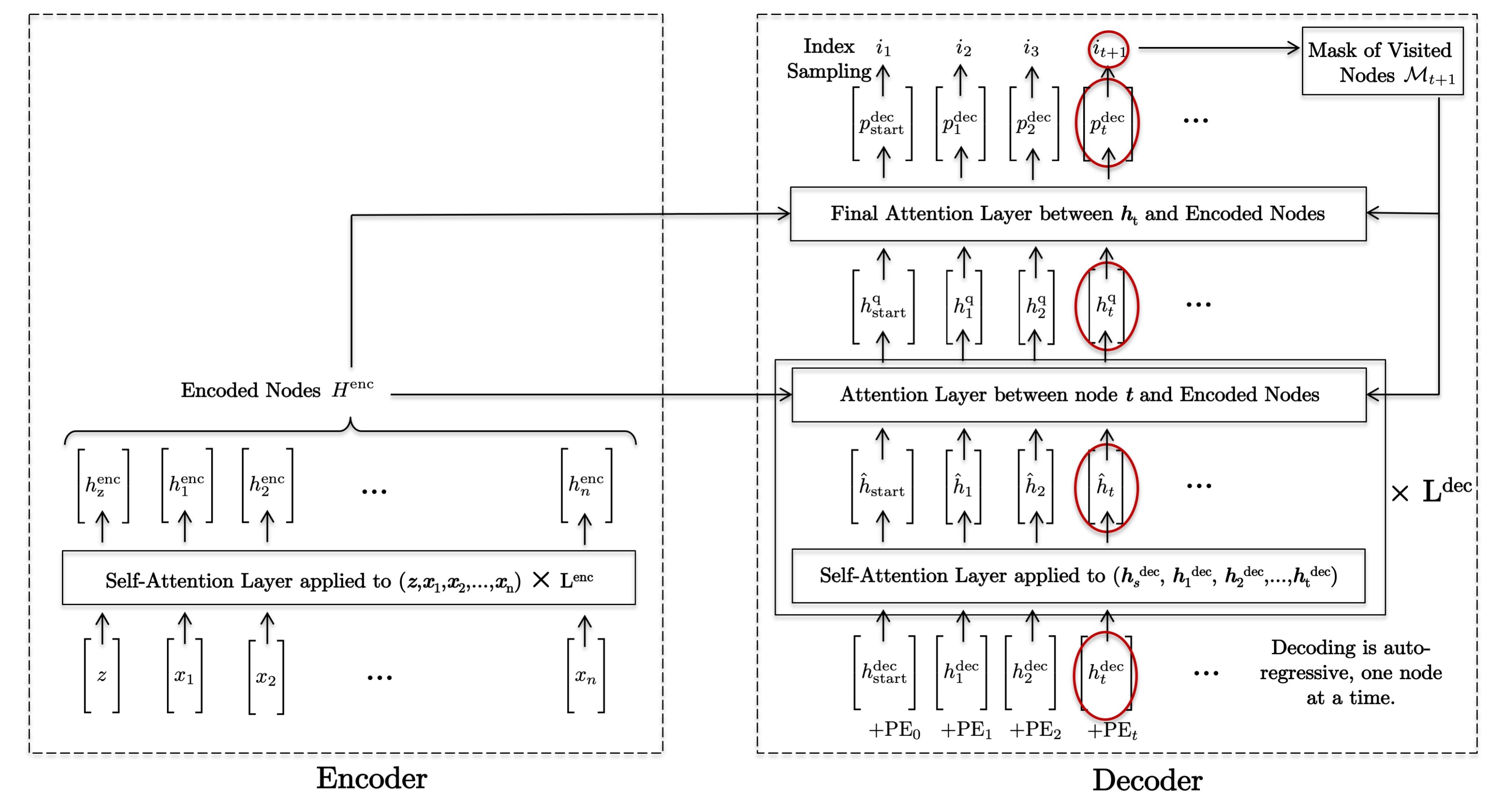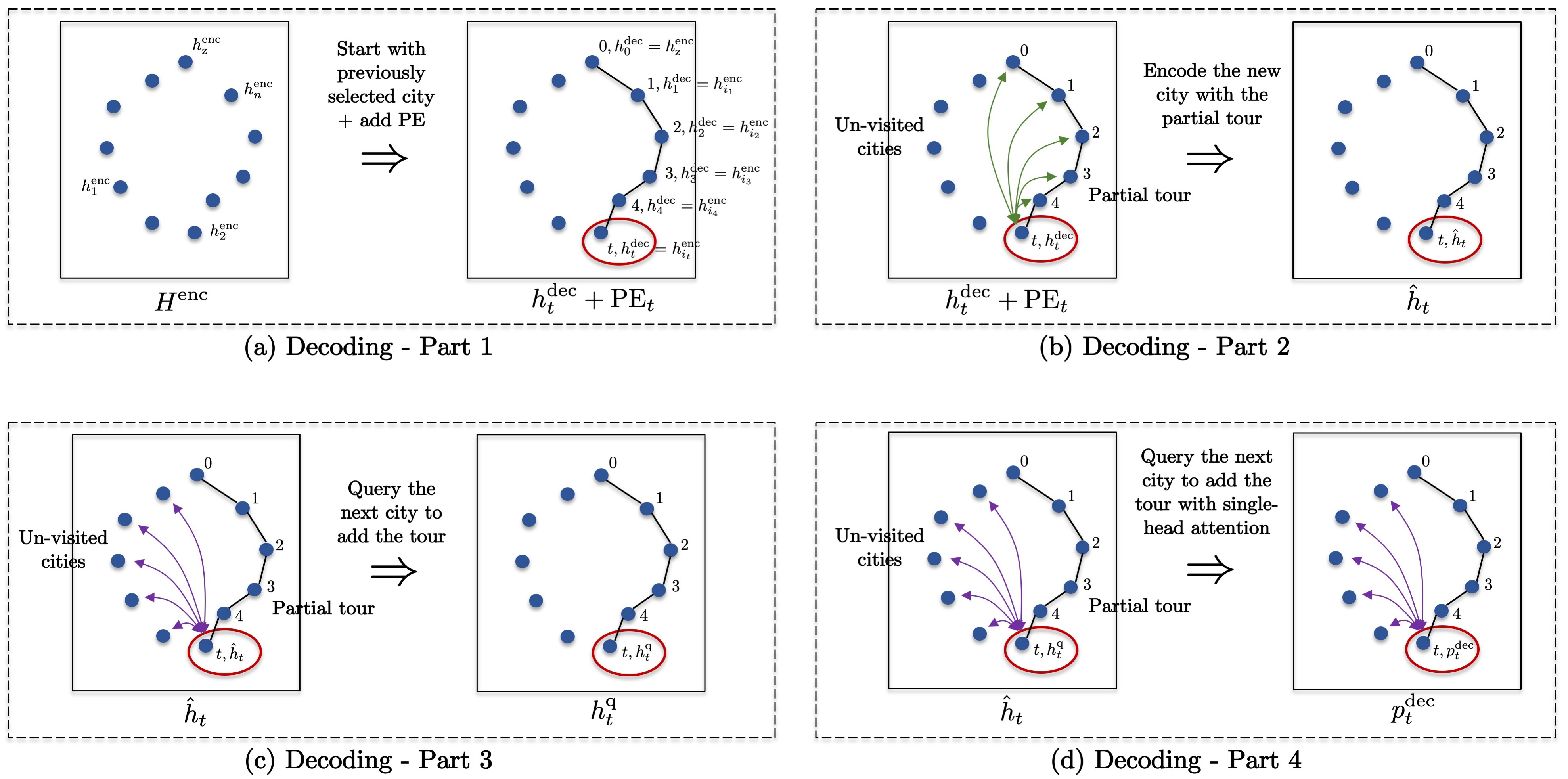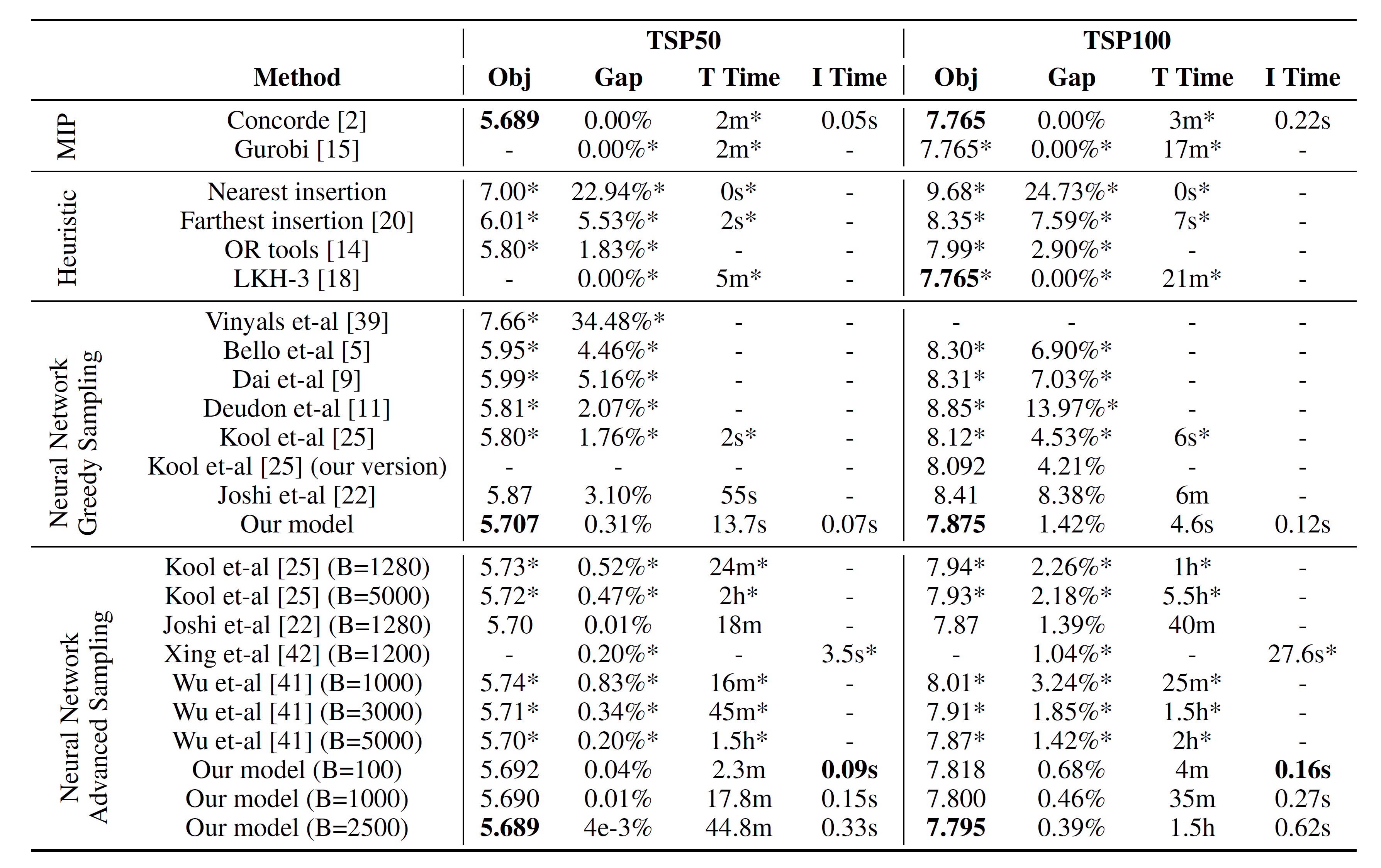# The Transformer Network for the Traveling Salesman Problem

Posted by MY on September 30, 2021

# The Transformer Network for the Traveling Salesman Problem

## 一、TSP的传统求解方式

• 精确算法：可以保证找到最优解，但是当问题规模很大时往往很难求解。这类算法主要包括动态规划（Dynamic Programming）算法以及整数规划类（Integer Programming, IP）算法
• 动态规划算法的求解复杂度为$\mathrm{O}\left(n^{2} 2^{n}\right)$，当$n>40$时往往非常难解
• Gurobi是一种较为通用的求解器，可以用于求解许多组合优化问题。它是基于整数规划算法以及Cutting Planes (CP) and Branch-and-Bound (BB)技巧而设计的
• Concorde是针对TSP定制的求解器，本质上也是基于IP+CP+BB设计的，是传统方法中最快的TSP求解器
• 近似/启发式算法（Approximate/Heuristic）：针对特定的问题，使用人工设计的启发式算法进行求解。这类算法牺牲了一些最优性，以换取较高的求解效率。经典的一些算法包括：
• Christofides算法使用最小生成树（Minimum Spanning Trees）来近似 TSP。 该算法的多项式时间复杂度为$\mathrm{O}(n^2 log n)$，并且可以保证找到的路径长度在最优解的1.5倍以内（以下称作近似最优比例，approximation ratio）
• Farthest/nearest/greedy insertion算法的时间复杂度为$\mathrm{O}(n^2)$，其中Farthest insertion的近似最优比例为2.43
• Google OR-Tools可以求解 TSP 和一系列的车辆路径规矩问题。 OR-Tools集成了多种启发式 算法，包括模拟退火（Simulated Annealing）、贪婪下降（ Greedy Descent）、禁忌搜索（Tabu Search）等，并通过局部搜索（Local Search）技术进一步进行优化。
• 2-Opt 算法做法为随机选取一对节点$i$和$k$，将$i$之前的路径不变添加到新路径中，将$i$到$k$之间的路径翻转其编号后添加到新路径中，将$k$之后的路径不变添加到新路径中。其复杂度为$\mathrm{O}(n^2m(n))$，其中 $n^2$是节点对的数量，$m(n)$ 是测试所有的节点对以达到局部最小值的必须次数（最坏情况为 $\mathrm{O}(2^{n/2})$)。 近似最优比例为$4 / \sqrt{n}$。后续工作沿用类似的思路提出了3-opt等方法。
• LKH-3 算法是目前求解 TSP 最好的启发式方法。它是基于 2-Opt/3-Opt 的原始 LKH 和LKH-2的扩展，使用最小生成树来筛选候选边进行不断的交换。

## 二、利用神经网络求解TSP

• HopfieldNets：第一个旨在解决小规模TSP 的神经网络。
• PointerNets：使用DL解决 TSP 和组合优化问题的先驱工作。这项工作将循环神经网络与注意力机制结合起来，对城市进行编码并解码（一次输出一个节点）以获得旅行商要访问的的节点序列。该网络的参数是采用监督学习的方式，通过最小化PointerNets得到的解与传统解法得到的解之间的差别来更新的。
• PointerNets+RL ：作者使用RL改进了传统的PointerNets，从而无需预先获取TSP的启发式解来作为监督训练数据的标签。其中，RL算法中的奖励值设置为了获得的解的长度。
• Order-invariant PointerNets+RL：PointerNets求解的结果会受到输入城市顺序排列的影响，而在TSP中我们其实希望求得的解在任意输入顺序下都能找到最优解，因此便需要网络对输入序列顺序保持不变性。工作  通过修改编码器的结构来达到这个目的。
• S2V-DQN：这个模型是一个图网络，它输入所有节点构成的图以及当前已构建的路径，并通过输出的状态值函数$Q$来选择路径的下一个节点。使用DQN算法进行训练。
• Transformer-encoder+2-Opt heuristic：作者使用标准的Transformer对城市进行编码，并使用当前已构建的路径中的最后三个城市组成的query来进行解码。该网络使用 Actor-Critic RL 进行训练，并使用标准的 2-Opt 启发式算法改进解。
• Transformer-encoder+Attention-decoder：这项工作也使用了一个标准的transformer来编码城市，解码所用的query则包括已构建路径中第一个和最后一个访问的城市以及所有城市的全局表征。使用REINFORCE算法来进行训练。
• GraphConvNet：这项工作通过监督学习的方式来学习一个图网络，以预测一条边在 TSP 最优路径中的概率，然后 通过Beam Search来生成可行的解。
• 2-Opt Learning：这项工作设计了一个基于 Transformer 的网络来学习为 2-Opt 启发式算法选择交互所用的节点。 使用Actor-Critic RL 进行训练。
• GNNs with Monte Carlo Tree Search：将蒙特卡洛树搜索（Monte-Carlo Tree Search, MCTS）与图网络结合，将单个节点候选的评估变为多个节点候选的评估。

## 三、方法设计$H^{\mathrm{enc}}=H^{\ell=L^{\mathrm{nc} c}} \in \mathbb{R}^{(n+1) \times d}$ \begin{aligned} H^{\ell=0} &=\operatorname{Concat}(z, X) \in \mathbb{R}^{(n+1) \times 2}, z \in \mathbb{R}^{2}, X \in \mathbb{R}^{n \times 2} \\ H^{\ell+1} &=\operatorname{softmax}\left(\frac{Q^{\ell} K^{\ell^{T}}}{\sqrt{d}}\right) V^{\ell} \in \mathbb{R}^{(n+1) \times d} \\ Q^{\ell} &=H^{\ell} W_{Q}^{\ell} \in \mathbb{R}^{(n+1) \times d}, W_{Q}^{\ell} \in \mathbb{R}^{d \times d} \\ K^{\ell} &=H^{\ell} W_{K}^{\ell} \in \mathbb{R}^{(n+1) \times d}, W_{K}^{\ell} \in \mathbb{R}^{d \times d} \\ V^{\ell} &=H^{\ell} W_{V}^{\ell} \in \mathbb{R}^{(n+1) \times d}, W_{V}^{\ell} \in \mathbb{R}^{d \times d} \end{aligned}

1. 获取上一次选择的节点$i_{t}$的表征：

\begin{aligned} h_{t}^{\mathrm{dec}} &=h_{i_{t}}^{\mathrm{enc}}+\mathrm{PE}_{t} \in \mathbb{R}^{d} \\ h_{t=0}^{\mathrm{dec}} &=h_{\mathrm{start}}^{\mathrm{dec}}=z+\mathrm{PE}_{t=0} \in \mathbb{R}^{d} \end{aligned}

其中PE为Transformer中标记节点顺序的positional embedding：

$\mathrm{PE}_{t, i}=\left\{\begin{array}{l} \sin \left(2 \pi f_{i} t\right) \text { if } i \text { is even, } \\ \cos \left(2 \pi f_{i} t\right) \text { if } i \text { is odd, } \end{array} \quad \text { with } f_{i}=\frac{10,000 \frac{d}{[2 i]}}{2 \pi}\right.$
1. 使用self-attention在已构建路径的上准备query：

\begin{aligned} \hat{h}_{t}^{\ell+1} &=\operatorname{softmax}\left(\frac{q^{\ell} K^{\ell^{T}}}{\sqrt{d}}\right) V^{\ell} \in \mathbb{R}^{d}, \ell=0, \ldots, L^{\mathrm{dec}}-1 \\ q^{\ell} &=\hat{h}_{t}^{\ell} \hat{W}_{q}^{\ell} \in \mathbb{R}^{d}, \hat{W}_{q}^{\ell} \in \mathbb{R}^{d \times d} \\ K^{\ell} &=\hat{H}_{1, t}^{\ell} \hat{W}_{K}^{\ell} \in \mathbb{R}^{t \times d}, \hat{W}_{K}^{\ell} \in \mathbb{R}^{d \times d} \\ V^{\ell} &=\hat{H}_{1, t}^{\ell} \hat{W}_{V}^{\ell} \in \mathbb{R}^{t \times d}, \hat{W}_{V}^{\ell} \in \mathbb{R}^{d \times d} \\ \hat{H}_{1, t}^{\ell} &=\left[\hat{h}_{1}^{\ell}, \ldots, \hat{h}_{t}^{\ell}\right], \hat{h}_{t}^{\ell}=\left\{\begin{array}{l} h_{t}^{\mathrm{dec}} \text { if } \ell=0 \\ h_{t}^{\mathrm{q}, \ell} \text { if } \ell>0 \end{array}\right.\\ \end{aligned}
2. 使用query在未访问的城市中查询下一个可能要访问的城市（已访问的城市使用$\mathcal{M}_{t}$进行mask，$\odot$是Hadamard product）：

\begin{aligned} h_{t}^{\mathrm{q}, \ell+1} &=\operatorname{softmax}\left(\frac{q^{\ell} K^{\ell^{T}}}{\sqrt{d}} \odot \mathcal{M}_{t}\right) V^{\ell} \in \mathbb{R}^{d}, \ell=0, \ldots, L^{\mathrm{dec}}-1 \\ q^{\ell} &=\hat{h}_{t}^{\ell+1} \tilde{W}_{q}^{\ell} \in \mathbb{R}^{d}, \tilde{W}_{q}^{\ell} \in \mathbb{R}^{d \times d} \\ K^{\ell} &=H^{\mathrm{enc}} \tilde{W}_{K}^{\ell} \in \mathbb{R}^{t \times d}, \tilde{W}_{K}^{\ell} \in \mathbb{R}^{d \times d} \\ V^{\ell} &=H^{\mathrm{enc}} \tilde{W}_{V}^{\ell} \in \mathbb{R}^{t \times d}, \tilde{W}_{V}^{\ell} \in \mathbb{R}^{d \times d} \end{aligned}
3. 获得未访问城市的概率分布：

\begin{aligned} p_{t}^{\mathrm{dec}} &=\operatorname{softmax}\left(C \cdot \tanh \left(\frac{q K^{T}}{\sqrt{d}} \odot \mathcal{M}_{t}\right)\right) \in \mathbb{R}^{n} \\ q &=h_{t}^{\mathrm{q}} \bar{W}_{q} \in \mathbb{R}^{d}, \bar{W}_{q} \in \mathbb{R}^{d \times d} \\ K &=H^{\text {enc }} \bar{W}_{K} \in \mathbb{R}^{n \times d}, \bar{W}_{K}^{\ell} \in \mathbb{R}^{d \times d} \end{aligned} 其中$C=10$

整体的四个步骤如图2所示：图2 decoding四个步骤示意图

$\max _{\operatorname{seq}_{n}=\left\{i_{1}, \ldots, i_{n}\right\}} P^{\mathrm{TSP}}\left(\operatorname{seq}_{n} \mid X\right)=P^{\mathrm{TSP}}\left(i_{1}, \ldots, i_{n} \mid X\right)$

$P^{\mathrm{TSP}}\left(i_{1}, \ldots, i_{n} \mid X\right)=P\left(i_{1} \mid X\right) \cdot P\left(i_{2} \mid i_{1}, X\right) \cdot P\left(i_{3} \mid i_{2}, i_{1}, X\right) \cdot \ldots \cdot P\left(i_{n} \mid i_{n-1}, i_{n-2}, \ldots, X\right)$

$\max _{i_{1}, \ldots, i_{n}} \Pi_{t=1}^{n} P\left(i_{t} \mid i_{t-1}, i_{t-2}, \ldots i_{1}, X\right)$

$\left\{i_{1}^{b}, \ldots, i_{t}^{b}\right\}_{b=1}^{B}=\text { Top-B }\left\{\Pi_{k=1}^{t} P\left(i_{k}^{b} \mid i_{k-1}^{b}, i_{k-2}^{b}, \ldots, i_{1}^{b}, X\right)\right\}_{b=1}^{B \cdot(n-t)}$

## 四、实验结果## 五、结论

• 本文的方法进一步说明了提取高效的表征并进行更好的采样会帮助基于学习的启发式算法更好地求解组合优化问题。
• 然而，当前基于学习的启发式算法距离传统的求解方式效果上还存在一定的差距；同时，当前的大部分求解方法都专注于规模较小的问题，在大规模的车辆路径规划问题上还鲜有探索。

## 参考

##### Share